# C 401: RSA with Very Small Keys (15 pts + 15 extra)

What you need:
• Any computer with Python 3.

## Purpose

To calculate RSA parameters and use them, in a very simple case with small numbers.

## RSA Calculations## 1. Choose Two Primes: p and q

Let's use p = 7 and q = 23

## 2. Compute n = p * q

Start Python 3 in interactive mode with this command:
``` python3 ```
Execute these commands to store p and q in the Python interpreter, and calculate n
```p = 7 q = 23 n = p * q print(p, q, n) ```
The parameters print out, as shown below. n = 161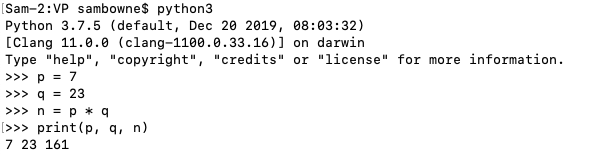## 3. Compute φ(n) = (p-1) * (q-1)

Execute these commands (I don't know how to use Greek in Python, so we'll use "phin" instead of φ(n)):
```phin = (p-1) * (q-1) print(p, q, n, phin) ```
The parameters print out, as shown below. phin = 132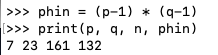## 4. Select Public Exponent e

e has to be between 1 and phin - 1 and it has to not share any divisors with phin. One simple way to meet these requirements is to pick a small prime number.

Let's use e = 5

## 5. Compute Private Key d

We need to find a d with this property:
(d * e) mod phin = 1
First let's set e and perform a simple multiplication.

Execute these commands. As shown below, 20 * 5 is 100, as you'd expect.

```e = 5 print(20 * e) ```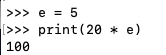Now let's use modular arithmetic, with a modulus of phin, that is, 132. In Python, we use a % character to indicate a modulus.

Modulus 132 means there is no number larger than 131. Adding 1 to 131 makes it roll back to 0. The numbers are on a ring, as shown below.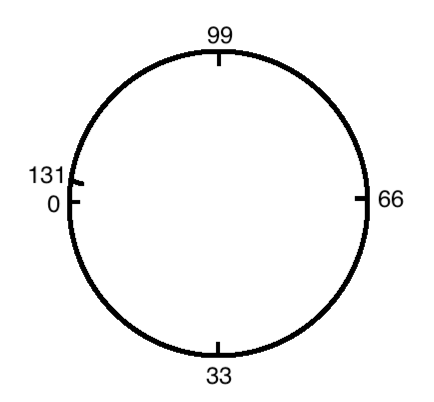Execute these commands. As shown below, 132 modulus phin is zero.

```print(100 % phin) print(130 % phin) print(131 % phin) print(132 % phin) ```Now let's calculate the result for values of d from 20 through 25.

Execute these commands:

```print((20 * e) % phin) print((21 * e) % phin) print((22 * e) % phin) print((23 * e) % phin) print((24 * e) % phin) print((25 * e) % phin) print((26 * e) % phin) print((27 * e) % phin) ```
We weren't able to find a good value for d -- 26 resulted in 130, and 27 resulted in 3, as shown below. We went right past 1.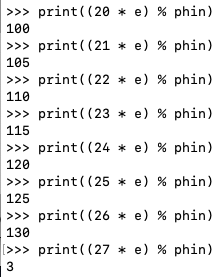We'll have to use larger values and try to hit 1 the next time we go around the ring.

Execute these commands:

```print((50 * e) % phin) print((51 * e) % phin) print((52 * e) % phin) print((53 * e) % phin) ```
We have a winner, as shown below! Let's use d = 53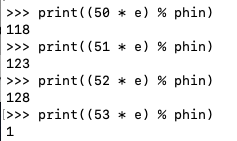## Encrypting a Message

Meghan wants to get private email, so she uses the values above to generate her key pair, as shown below.

She publishes her public key to the cloud.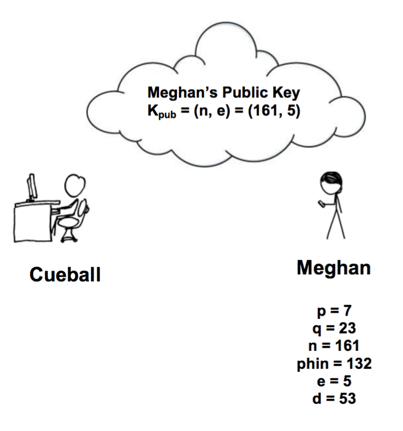Cueball wants to send Meghan this message:

```Hi! ```
We can only send numbers. Let's encode that message with ASCII using the table below.

So the message is:

```72 105 33 ```
A public key contains two numbers: n and e. To encrypt a message x, use this formula:Execute these commands:

```print(72 ** e % n) print(105 ** e % n) print(33 ** e % n) ```
As shown below, the encrypted message is 151 119 157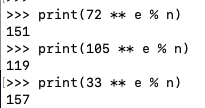## Decrypting the Message

To decrypt a message, use this formula:Execute these commands:
```d = 53 print(151 ** d % n) print(119 ** d % n) print(157 ** d % n) ```
As shown below, the decrypted message is 72 105 33 or Hi!.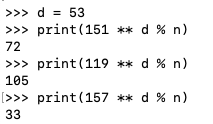# C 401.1: Cueball's Keys (15 pts)

Cueball will use these values:
```p = 13 q = 41 e = 7 ```
Calculate Cueball's public and private keys.

The flag is Cueball's Public Key, like this: (161,5)

# C 401.2: Message to Cueball (15 extra)

Meghan sends this message to Cueball. Decrypt it.

460, 364, 144, 153, 501, 402, 19, 311, 501, 280, 501, 80, 153, 382, 296, 153, 311

Hint: use the chr() and ord() functions

The flag is the decrypted message.

## Sources

Cracking short RSA keys
Cueball image
Megan image
Cloud image

Posted 3-23-16 by Sam Bowne